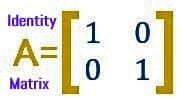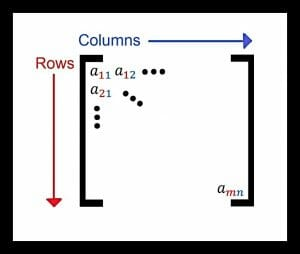# Matrices

When most people think of the word “matrix,” they probably think of the 1999 movie starring Keanu Reeves. The movie only relates to the mathematical concept of matrices insofar as the sinister computers in the movie use matrices to operate, as many real-life computers do.Indeed, matrices do have applications in computer science because they are a convenient and compact way to represent large sets of numbers. Some economic theories can also be represented well with matrices. In mathematics, the principles of matrices are essential for graph theory and real analysis.

This guide begins by considering small matrices, namely ones with two columns and two rows.

The topic begins by detailing how to describe them and do operations on 2×2 matrices. It also explains how these concepts can be used to represent information.

The second topic in this guide generalizes the information from the first part to all matrices. It ends by introducing row reduction strategies for solving matrices and a few other more advanced topics that are used in other branches of mathematics.

## Introduction to 2×2 Matrices

A 2×2 matrix has two rows and two columns. They do have some similarities to tables insofar as they have the rows and columns, but there are crucial differences. Tables are meant to be a graphical display. They can be manipulated to show information in certain lights, but operations between tables makes no sense.

On the other hand, matrices are an array of a set of numbers. There are no hard divisions between numbers as there are in a table, and there are ways to perform operations using matrices.Operations with matrices, however, are more involved than the basic mathematical operations used for adding, subtracting, multiplying, and dividing individual terms or even functions.

This is partially due to the fact that a matrix typically contains many elements (also known as entries), and operations are usually done with other matrices except in the case of scalar multiplication. It is possible, however, to add, subtract, multiply, and take powers of matrices with certain restrictions. It is also possible to find the inverse of some matrices.

This topic introduces these concepts with 2×2 matrices before generalizing them for matrices of any size in the next section.

It opens with a description of matrices and then introduces the operations of addition, subtraction, scalar multiplication, and matrix multiplication. It then discusses the 2×2 identity matrix, shown above, and its properties. The section next deals with determinants and how they can be used to find whether a matrix has an inverse and, if so, what it is.

Finally, the topic concludes with ways that matrices can be used mathematically to represent information.

• Describing Matrices
• Equal Matrices
• Types of Matrices
• Scalar Multiplication
• Matrix Multiplication
• Identity Matrix
• Determinant of a Matrix
• Inverse of a Matrix
• Singular Matrix
• Solving a System of Equations
• Representing Information

## Introduction to Other Matrices

The concepts introduced in the first topic for 2×2 matrices can be extended to other matrices. Technically, a matrix can have infinitely many rows and columns, and a matrix with m rows and n columns is called an m x n matrix (read “m by n”). Representations of a generic matrix usually include elements with two subscripts.

The first represents the row of the element and the second represents the column of the element.

For example, the first element in the upper left of a generic matrix would have the subscript 11, while the one in the bottom right of an m x n matrix would have the subscript mn, as shown in the picture.Matrices used to represent information in subjects such as computer science can be huge, even though they are actually a condensed and convenient representation.

Although things like matrix multiplication involve a lot of steps, there are repetitive algorithms that programs can use to calculate large-scale matrix multiplication.

This section begins by generalizing the introduction to matrices and matrix operations. It then discusses how to find determinants of 2x2and 3×3 before generalizing the process for any square matrix.

This information is used to explain how to simplify and find the inverse of such matrices. The topic also covers how the inverse can be used to solve systems of equations represented using matrices. Finally, the topic concludes by explaining row reduction methods and other applications of matrices.

• Introduction to Matrices
• Matrix Scalar Multiplication
• Matrix Multiplication
• Identity Matrix
• Determinant of a 2×2 Matrix
• Determinant of a 3×3 Matrix
• Simplifying the Determinant
• Inverse of a 2×2 Matrix
• Inverse of a 3×3 Matrix
• Singular Matrix
• Solving a 2×2 System of Equations Using a Matrix Inverse
• Solving a 3×3 System of Equations Using a Matrix Inverse
• Using Gauss-Jordan Method to Solve a System of Three Linear Equations
• Row Reducing a Matrix to Solve a System of Equations
• Solving a System of Equations using Matrix Row Transformations
• Cramer’s Rule
• Using Determinant to Find the Area of a Parallelogram
• Using Determinant to find the Area of a Triangle and a Polygon